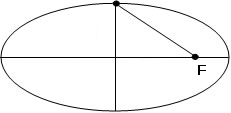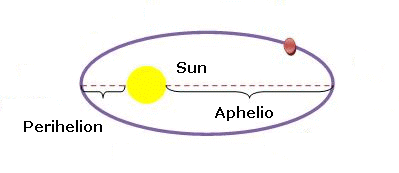### Ellipse

The ellipse is a closed curve figure, where the sum of the distances from two fixed points (foci) to every point on the curve is constant.
The foci are the two fixed points on the interior of an ellipse used in the formal definition of the ellipse.
Definitions :
Major half Axis : the half larger diameter of an ellipse;
Minor half Axis : the half smaller diameter of an ellipse;
Perimeter or circumference : total length of curve ellipse ;
Area : the ellipse area;
Eccentricity : a measure of how close is from a circle curve;
Foci distance : distance between the foci of the ellipse;
Y/X ratio , Minor Axis / Major Axis , a height to width ratio: A measure of eccentricity. An ellipse whose ratio of the minor axis to the major is 1: 1 can be called the circle.
The planets describe elliptical orbits around the sun, which is located in a focus of the orbital ellipse, so, Perihelion is the closest point to the sun, while Aphelion is the furthest point.
Enter data using the point as a decimal separator. Example: 1250.37; the results will be shown after a click on Calculate.Ellipse Decimal places: Major half Axis: Minor half Axis: Area: Perimeter (approx) Area: Eccentricity: Minor axis / Major axis: Foci distance: Major half axis: Minor half axis: Average distance: Perihelion: Aphelion:

Note:The accuracy of the calculator and its applicability to particular cases is not guaranteed.